# List of Geometry Formulas: The Super Complete List 2020

Geometry is one of the most essential and important field of Mathematics, and it has applications in almost every field. However, just like any other sub-field of mathematics, it involves a lot of formulas. So, having a list of geometry formulas in one place is a great accessory.

Specially, in exam days, these lists help students to memorize all the formulas very easily ( We don’t suggest you use them as cheating material).

With that in mind, we have compiled a list of all geometry formulas, for almost all of the properties. From area to perimeter to volume to angles and beyond. Also, we have included a short definition of all the shapes as well.

The list will be categorized according to types of shapes, that is first 2D shapes like Square and Circle etc. and then 3D Shapes like Cube and Sphere etc.

So, let’s get on with it.

## Two Dimensional (2D) Shapes

### SQUARE

Square is a quadrilateral (having four sides) whose all sides are equal in length and all sides are perpendicular (at 90 Degrees) to their adjacent side.

AREA: (Length Side) X (Length of Side) PERIMETER: 4 X ( Length of Side)

### Rectangle

In a rectangle, unlike square only the parallel sides are equal in length. All four angles are still of 90 Degrees.

Area of Rectangle = (Length) x (Width) Perimeter of Rectangle = 2 (Length + Width)

### Triangle

A Triangle has three sides and hence, three internal angles.

Triangles are of six types, namely Equilateral Triangle, Right Angled Triangle, Isosceles Triangle, Scalene Triangle, Acute Triangle and Obtuse Triangle.

Equilateral Triangle has all three sides of equal lengths, and hence all internal angles are equal, that is of 60 Degrees.

Right Angled Triangle has atleast one angle of 90 Degrees.

Isosceles Triangle has two sides of equal length and one of different length of other two.

Scalene Triangle has three sides of unequal lengths.

Acute Triangle has all three angles less than 90 Degrees.

Obtuse Triangle has two acute angles (less than 90) and one obtuse angle (greater than 90).

#### Right Angled Triangles

For Right Angled Triangle, calculating three sides and angles is very simple, by using a formula called as Pythagoras Theorem. However, this formula is only applicable for right angled triangles.

For applying this theorem certain following terminologies need to be understood:

1. Side in front of 90 Degree Angle is called as Hypotenuse (h).
2. Side adjacent to 90 Degree Angle and in front of angle involved in the formula, which will be denoted by “a”, is called as Perpendicular (p).
3. Third side adjacent to angle involved in the formula is called as base (b).

Now, Pythagoras Theorem for Right Angled Triangles states that: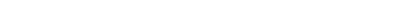Other Formulas for Right Angled Triangles are called as Trigonometric Ratios. They are: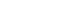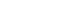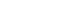#### Area, Base, Height and Altitude of Triangles

Base of a triangle is the bottom side of a triangle.

Altitude is a line that is perpendicular to the base and touches the vertix opposite of the base. Length of Altitude is called as Height of Triangle.

Now, knowing these terminologies, area of a triangle can be calculated by: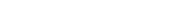### Circle

A circle can be defined as a shape with collection of points, which all are at same distance from another fixed point. That fixed is called Center of Circle.

Distance from Center to any point is called radius, and twice of radius is called diameter.

Overall perimeter of circle is called as Circumference.

Geometry Formulas for Circle are: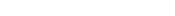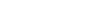### Parallelogram

A Parallelogram is a flat shape with opposite sides parallel and equal in length

Geometry Formulas are:

Area = b x h Perimeter = 2(b+s)

### Trapezium

Trapezium is a four sided shape whose only two opposite sides of the four are parallel. Other two are non-parallel.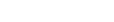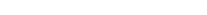So, now that was the geometry formulas list for 2D shapes.

Next up, 3D Shapes.

## Three Dimensional (3D) Shapes

### Cube

A cube is actually a 3D version of a square. It has six faces and all are of equal area. Length and Width of all six faces is also equal.

It’s Formulas are:

Volume = Side x Side x Side Surface Area = 6 x (Side x Side)

### Sphere

Sphere is a collection of points in 3 dimensions (unlike circle which is in 2), which all are at same distance (r) from a central point, called as the center of sphere.

Geometry Formulas for a sphere are: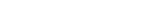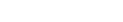### Cylinder

Cylinder is a shape that has two parallel circular bases, connected by a curvature face.

Geometry Formulas for Cylinder are: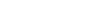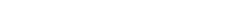## Wrapping Up

So, these were all the essential geometrical shapes and their formulas.

And one more thing, Learning Geometry in an interactive manner is a great and really fruitful experience. If you are interested in learning geometry online in best possible way. I recommend this interactive course from The Great Courses. You can watch it on Amazon Prime or buy a DVD. Link is below:

Share the article, if you liked it and it helped you.

How to Take Good Notes for College or School

How to Write a Research Proposal

How to Write References Page in Thesis and Assignments

1 comment

#### 1 comment

April 28, 2020 - 3:51 am

[…] ALSO CHECK OUT: List of All Geometry Formulas […]